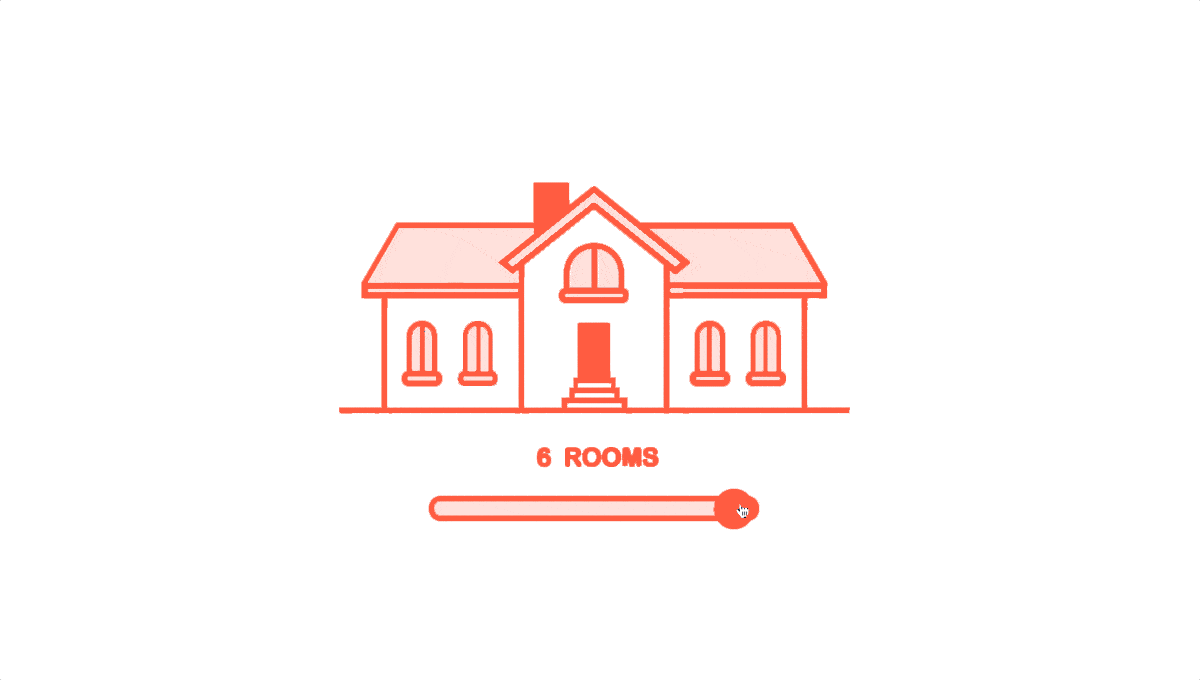If you use image as background than add class `.bg-img` in image and add below class in parent.

## ratio - "ratio2_3"

Add this class "ratio2_3" for this type of ratio

## ratio - "ratio_square"

Add this class "ratio_square" for this type of ratio

## ratio - "ratio_portrait"

Add this class "ratio_portrait" for this type of ratio

Light Dark
LTR RTL